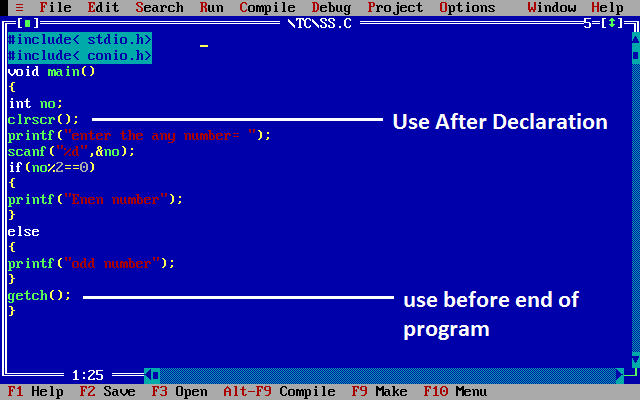# Clrscr() and Getch() in C

## Clrscr() and Getch() in C

clrscr() and getch() both are predefined function in "conio.h" (console input output header file).

## Clrscr() Function in C

It is a predefined function in "conio.h" (console input output header file) used to clear the console screen. It is a predefined function, by using this function we can clear the data from console (Monitor). Using of clrscr() function in C is always optional but it should be place after variable or function declaration only.

## Example of clrscr() Function in C

```#include<stdio.h>
#include<conio.h>

void main()
{
int a=10, b=20;
int sum=0;
clrscr();  // use clrscr() after variable declaration
sum=a+b;
printf("Sum: %d",sum);
getch();
}
```

Sum: 30

## Getch() Function in C

It is a predefined function in "conio.h" (console input output header file) will tell to the console wait for some time until a key is hit given after running of program.

By using this function we can read a character directly from the keyboard. Generally getch() function in C are placing at end of the program after printing the output on screen.

## Example of getch() Function in C

```#include<stdio.h>
#include<conio.h>

void main()
{
int a=10, b=20;
int sum=0;
clrscr();
sum=a+b;
printf("Sum: %d",sum);
getch();  // use getch() befor end of main()
}
```

## Output

Sum: 30